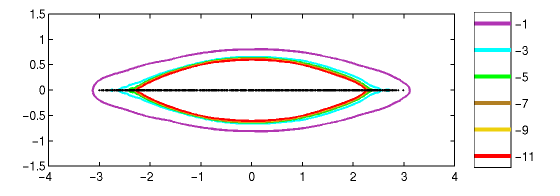Home | Introduction | Software | Examples | History | People | Bibliography | Applications | Theorems

## Pseudospectra of a Non-Hermitian Anderson Model Dimension N=1000This example of a Non-Hermitian Anderson model is a tridiagonal matrix with has exp(0.4) on the first super-diagonal, exp(-0.4) on the first sub-diagonal, and random entries uniformly distributed between [-1.5,1.5] on the main diagonal. This type of model was first suggested by N. Hatano and D. R. Nelson, "Localization transitions in non-Hermitian quantum mechanics", Phys. Rev. Lett. 77 (1996), 570-573. Pseudospectra of a related random bidiagonal model are analyzed in [TCE01].

Pseudospectra of Random Matrices: The Non-Hermitian Anderson Model.

Use the following MATLAB code compute a similar image using EigTool. To mimic the example above, set `N=1000` below.

```   N = 100;
g = 0.4;
A = exp(-g)*diag(ones(N-1,1),-1) + ...
diag(3*rand(N,1)-1.5) + ...
exp(g)*diag(ones(N-1,1),1);
opts.npts = 20;
opts.ax = [-4 4 -1.5 1.5];
opts.levels = [-11:-1];
eigtool(A, opts)
```